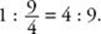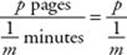﻿ ﻿Exercises - Ratios and Proportions - TOPICS IN ARITHMETIC - SAT SUBJECT TEST MATH LEVEL 1

## TOPICS IN ARITHMETIC## CHAPTER 4 Ratios and Proportions### Exercises1. If the ratio of teachers to administrators on a committee is 3 : 5, what percent of the committee members are administrators?

(A) 37.5%

(B) 40%

(C) 60%

(D) 62.5%

(E) It cannot be determined from the information given.

2. If the ratio of the measures of the two acute angles in a right triangle is 3 : 7, what is the degree measure of the smallest angle in the triangle?

(A) 9

(B) 18

(C) 27

(D) 36

(E) 54

3. If the ratio of the diameter of circle I to the diameter of circle II is 2 : 3, what is the ratio of the area of circle I to the area of circle II?

(A) 1 : 3

(B) 2 : 3

(C) 2 : 5

(D) 3 : 5

(E) 4 : 9

4. What is the ratio of the circumference of a circle to its radius?

(A) 1

(B)(C)(D) π

(E) 2π

5. If x > 0 and, then x =

(A) 3

(B) 9

(C) 27

(D) 36

(E) 72

6. If a varies inversely with b, and a = 3m when b = 5n, what is b when a = 5m?

(A)(B)(C) 3m

(D) 3n

(E) 5n

7. Jeremy can read 36 pages per hour. At this rate, how many pages can he read in 36 minutes?

(A) 3.6

(B) 21.6

(C) 43.2

(D) 72

(E) 1,296

8. If x varies directly with y2, and if x = 50 when y = 5, what is the value of x when y = 10?

(A) 5

(B) 25

(C) 50

(D) 100

(E) 200

9. Meri can type p pages inminutes. At this rate, how many pages can she type in m minutes?

(A) mp

(B) pm2

(C)(D)(E)1. (D) 4. (E) 7. (B) 2. (C) 5. (B) 8. (E) 3. (E) 6. (D) 9. (B)

Solutions

Each of the problems in this set of exercises is typical of a question you could see on a Math 1 test. When you take the model tests in this book and, in particular, when you take the actual Math 1 test, if you get stuck on questions such as these, you do not have to leave them out—you can almost always answer them by using one or more of the strategies discussed in the “Tactics” chapter. The solutions given here do not depend on those strategies; they are the correct mathematical ones.

See Important Tactics for an explanation of the symbol ⇒, which is used in several answer explanations.1. (D) Of every 8 committee members, 3 are teachers and 5 are administrators. Therefore, administrators make upof the committee.

2. (C) The sum of the measures of the two acute angles in a right triangle is 90°. So 3x + 7x = 9010x = 90x = 9. So the measure of the smaller acute angle is 3(9) = 27°.

3. (E) If the diameters of the two circles are 2x and 3x, their radii are x andSo their areas are πx2 andTherefore, the ratio of their areas is4. (E) By definition, π is the ratio of the circumference to the diameter of a circle, so5. (B) Cross multiply: (x – 3)(x + 3) = 72x2 – 9 = 72x2 = 81x = 9.

6. (D) If a varies inversely as b, there is a constant k such that ab = k. So (3m)(5n) = kk = 15mn. Then if a = 5m:7. (B) Set up a proportion:and cross multiply:8. (E) Since x varies directly with y2, there is a constant k such that. ThenSo when y = 10:9. (B) Meri types at the rate ofpages per minute = mp pages per minute. Since she can type mp pages each minute, in m minutes she can type m(mp) = pm2 pages.

﻿